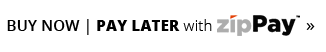0

Your Cart is Empty

# Edvantage - Multiplication MagicThe Multiplication Magic cards can be used effectively by the teacher as a teaching aid or by individual students to learn and revise the multiplication and corresponding division facts from 1 times to 12 times. The teacher can ask the class to read the multiplication sentence as a card is held up ( Eg: 6 x 4 = 24, 4 x 6 = 24) or alternatively cover the numeral at the top of the card (the product) to see how quickly the children can provide the answer.

The simple design allows individual students to work independently as they learn and memorise the multiplication and division facts and complete the revision exercise on the back of each card, using a whiteboard marker on the write-on /wipe-off surface. The cards are colour coded to indicate the level of difficulty. Contains 76 sturdy laminated cards measuring 12.3cm x 12.3cm in a durable storage tine.

Multiplication
Multiply the two bottom numbers. The answer (product) is at the top. 1 x 2 = 2 2 x 1 = 2.
Division
Start with the number at the top. Divide it by a number at the bottom. Cover the selected number. The answer will be the remaining bottom number. 2 / 1 =2 2 / 2 = 1.

3 Years +